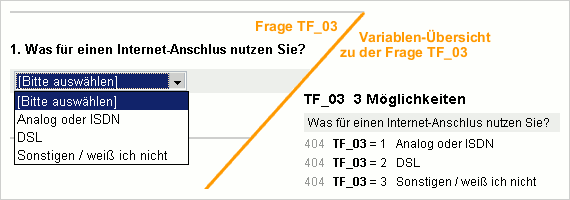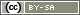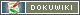# Conditions with More Than 2 Options

How the questionnaire continues after a filter is often not just limited to two options; in fact there are several possibilities as to how it progresses. For example, people with an analog internet connection or ISDN should be asked about the tariff they use; DSL users about the speed and connection provider. People who have a different connection will get questions about the type of cable between the computer and the wall.

Two different language constructs can be used in such cases: `elseif` and `switch`. The former is linked to the well-known if-else construct; the latter is explained here more for the sake of completeness. Occasionally, using `switch` is more concise if several values of the same variable should be checked.

## Nested IF and ELSE

If-then-else filters can be extended indefinitely.:

• If the internet connection is analog or ISDN, then question 1 is asked.
• If not, then a new condition is checked:
• If the connection is DSL, then question 2 is asked.
• If not, then a new condition is checked…
• This sequence can be extended indefinitely.If question TF03 regarding the internet connection has been put on page 1, then the filter on page 2 in PHP code looks as follows:

```if (value('TF03') == 1) {  // analog or ISDN?
} else {
// in the 'else' part there is a new filter (nested)
if (value('TF03') == 2) {  // DSL connection?
question('IN16');  // question on DSL connection
} else {
// in the 'else' part there is a new filter (nested)
if (value('TF03') == 3) {  // other connection?
question('IN17');  // question on cabel
} else {
html('<p>You have not given an answer!</p>');
}
}
}```

These nested constructions can be useful – but in this example it also works more concisely.

## Continue IF and ELSE

The following construction returns the exact same result as in the previous example. It can also be extended indefinitely.

```// example for else if - not nested
if (value('TF03') == 1) {  // analog oder ISDN?
} else if (value('TF03') == 2) {  // DSL connection?
question('IN16');  // question on DSL connection
} else if (value('TF03') == 3) {  // other connection?
question('IN17');  // question on cable
} else {
html('<p>You have not given an answer!</p>');
}```

## ELSE + IF = ELSEIF

The `elseif` command in PHP can be used to simplify this. The statement after this command is then executed if the condition after “elseif” is true, but none of the conditions preceding it are. In practice, `elseif` is no different to the `else if` in the example above.

```// PHP code – example for elseif
if (value('TF03') == 1) {  // analog or ISDN?
} elseif (value('TF03') == 2) {  // DSL connection?
question('IN16');  // question on DSL connection
} else {  // none of the previous?
// if a question response was required for the question
// this saves checking the last condition
question('IN17');  // question on cable
}```

The value from TF03 can also be saved in advance in a variable (PHP Variables). This makes it easier again:

```// save value in variable
\$cn = value('TF03');
if (\$cn == 1) {        // analog or ISDN?
} elseif (\$cn == 2) {  // DSL connection?
question('IN16');      // question on DSL connection
} else {                 // none of the previous?
question('IN17');      // question on cable
}```

## SWITCH

If, as in the example, only different values of a variable should be called up, it is obviously somewhat long-winded to write `value('TF03')` each time. Instead of doing this, the `switch` construction in PHP can be used.

To do this, the value to be checked is stated in brackets behind the `switch`. The different options are written after each `case`, followed by a colon.

Note: There must always be a `break` after the command (in contrast to the `elseif` construction) – otherwise everything from `case` onwards will be executed.

```switch (value('TF03')) {
case 1:
break;
case 2:
question('IN16');  // question on DSL connection
break;
case 3:
question('IN17');  // question on cable
break;
default:             // default used, if no case applies
break;             // the last break is not necessary
}
// other questions which all respondents are asked
question('BB01');```

Of course, this can be written in such a way to take up less space:

```switch (value('TF03')) {
case 1: question('IN15'); break;  // question about tariff
case 2: question('IN16'); break;  // question on DSL connection
case 3: question('IN17'); break;  // question on cable